# SUMIF in Google Sheets with formula examples

The tutorial shows how to use the SUMIF function in Google spreadsheets to conditionally sum cells. You will find formula examples for text, numbers and dates and learn how to sum with multiple criteria.

Some of the best functions in Google Sheets are those that help you summarize and categorize data. Today, we are going to have a closer look at one of such functions - SUMIF - a powerful instrument to conditionally sum cells. Before studying the syntax and formula examples, let me begin with a couple of important remarks.

Google Sheets has two functions to add up numbers based on conditions: SUMIF and SUMIFS. The former evaluates just one condition while the latter can test multiple conditions at a time. In this tutorial, we will focus solely on the SUMIF function, the use of SUMIFS will be covered in the next article.

If you know how to use SUMIF in Excel desktop or Excel online, SUMIF in Google Sheets will be a piece of cake for you since both are essentially the same. But don't rush to close this page yet - you may find a few unobvious but very useful SUMIF formulas you didn't know!

## SUMIF in Google Sheets - syntax and basic uses

The SUMIF function is Google Sheets is designed to sum numeric data based on one condition. Its syntax is as follows:

SUMIF(range, criterion, [sum_range])

Where:

• Range (required) - the range of cells that should be evaluated by criterion.
• Criterion (required) - the condition to be met.
• Sum_range (optional) - the range in which to sum numbers. If omitted, then range is summed.

As an example, let's make a simple formula that will sum numbers in column B if column A contains an item equal to the "sample item".

For this, we define the following arguments:

• Range - a list of items - A5:A13.
• Criterion - a cell containing the item of interest - B1.
• Sum_range - amounts to be summed - B5:B13.

Putting all the arguments together, we get the following formula:

`=SUMIF(A5:A13,B1,B5:B13)`

And it works exactly as it should: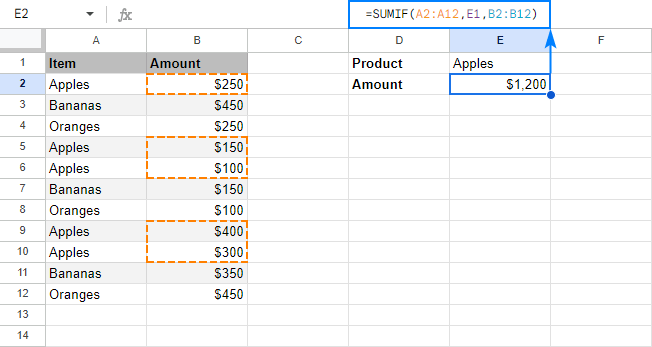From the above example, you may have the impression that using SUMIF formulas in Google spreadsheets is so easy that you could do it with your eyes shut. In most cases, it is really so :) But still there are some tricks and non-trivial uses that could make your formulas more effective. The below examples demonstrate a few typical use cases. To make the examples easier to follow, I invite you to open our sample SUMIF Google Sheet.

### SUMIF formulas with text criteria (exact match)

To add up numbers that have a specific text in another column in the same row, your simply supply the text of interest in the criterion argument of your SUMIF formula. As usual, any text in any argument of any formula should be enclosed in "double quotes".

For example, to get a total of bananas, you use this formula:

`=SUMIF(A5:A13,"bananas",B5:B13)`

Or, you can put the criterion in some cell and refer to that cell:

`=SUMIF(A5:A13,B1,B5:B13)`

This formula is crystal clear, isn't it? Now, how do you get a total of all items except bananas? For this, use the not equal to operator:

`=SUMIF(A5:A13,"<>bananas",B5:B13)`

If an "exclusion item" is input in a cell, then you enclose the not equal to operator in double quotes ("<>") and concatenate the operator and cell reference by using an ampersand (&). For example:

`=SUMIF (A5:A13,"<>"&B1, B5:B13)`

The following screenshot demonstrates both "Sum if equal to" and "Sum if not equal to" formulas in action: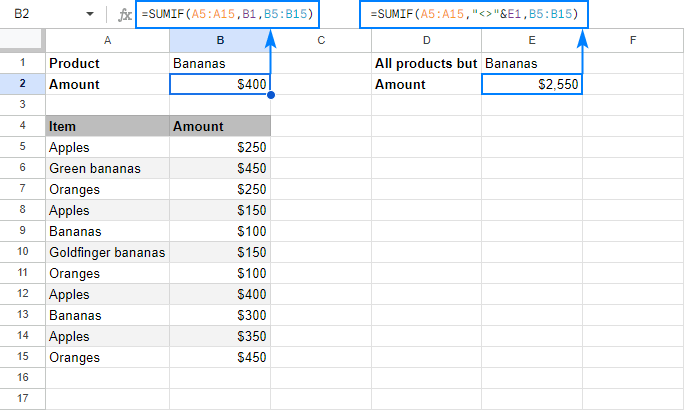Please note that SUMIF in Google Sheets searches for the specified text exactly. In this example, only Bananas amounts are summed, Green bananas and Goldfinger bananas are not included. To sum with partial match, use wildcard characters as shown in the next example.

### SUMIF formulas with wildcard characters (partial match)

In situations when you want to sum cells in one column if a cell in another column contains a specific text or character as part of the cell contents, include one of the following wildcards in your criteria:

• Question mark (?) to match any single character.
• Asterisk (*) to match any sequence of characters.

For example, to sum the amounts of all sorts of bananas, use this formula:

`=SUMIF(A5:A13,"*bananas*",B5:B13)`

You can also use wildcards together with cell references. For this, enclose the wildcard character in quotation marks, and concatenate it with a cell reference:

`=SUMIF(A5:A13, "*"&B1&"*", B5:B13)`

Either way, our SUMIF formula adds up the amounts of all bananas: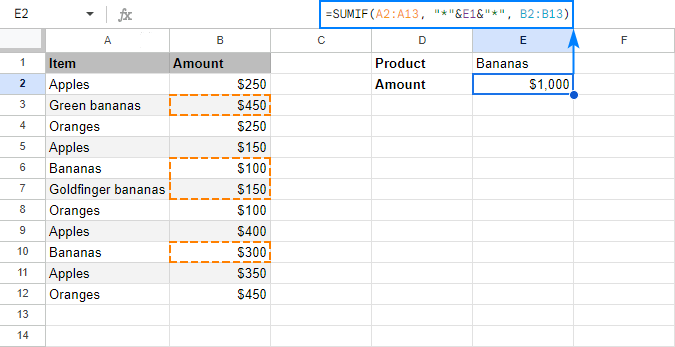To match an actual question mark or asterisk, prefix it with the tilde (~) character like "~?" or "~*".

For example, to sum numbers in column B that have an asterisk in column A in the same row, use this formula:

`=SUMIF(A5:A13, "~*", B5:B13)`

You can even type an asterisk in some cell, say B1, and concatenate that cell with the tilde char:

`=SUMIF(A5:A13, "~"&B1, B5:B13)`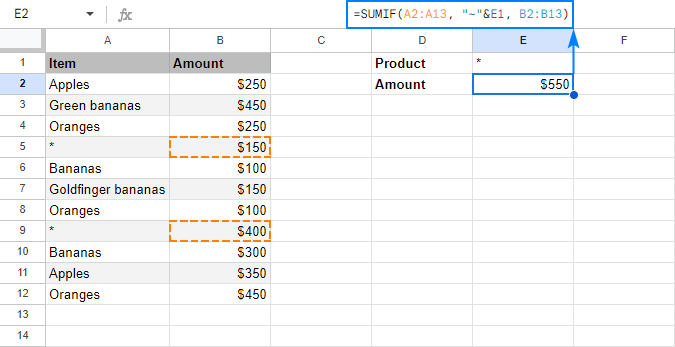### Case-sensitive SUMIF in Google Sheets

By default, SUMIF in Google Sheets does not see the difference between small and capital letters. For force it to teat uppercase and lowercase characters differently, use SUMIF in combination with the FIND and ARRAYFORMULA functions:

SUMIF(ARRAYFORMULA( FIND("text", range)), 1, sum_range)

Supposing you have a list of order numbers in A5:A13 and corresponding amounts in C5:C13, where the same order number appears in several rows. You enter the target order id in some cell, say B1, and use the following formula to return the order total:

`=SUMIF(ARRAYFORMULA(FIND(B1, A5:A13)),1, C5:C13)`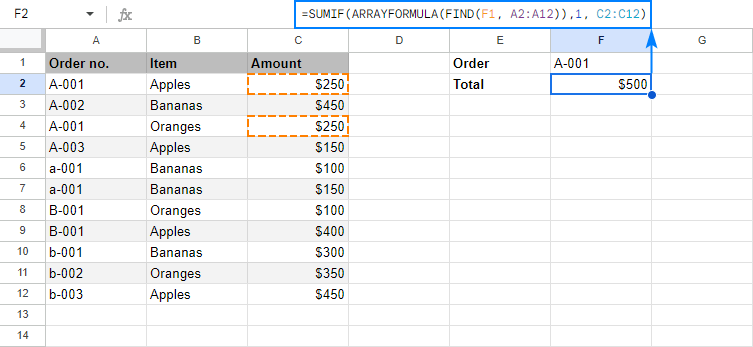#### How this formula works

To better understand the formula's logic, let's break it down into the meaningful parts:

The trickiest part is the range argument: ARRAYFORMULA(FIND(B1, A5:A13))

You use the case-sensitive FIND function to look for the exact order id. The problem is that a regular FIND formula can only search within a single cell. To search within a range, an array formula is needed, so you nest FIND inside ARRAYFORMULA.

When the above combination finds an exact match, it returns 1 (the position of the first found character), otherwise a #VALUE error. So, the only thing left for you to do is to sum the amounts corresponding to 1's. For this, you put 1 in the criterion argument, and C5:C13 in the sum_range argument. Done!

### SUMIF formulas for numbers

To sum numbers that meet a certain condition, use one of the comparison operators in your SUMIF formula. In most cases, choosing an appropriate operator is not a problem. Embedding it in the criterion properly could be a challenge.

#### Sum if greater than or less than

To compare the source numbers to a particular number, use one of the following logical operators:

• greater than (>)
• less than (<)
• greater than or equal to (>=)
• less than or equal to (<=)

For example, to add up numbers in B5:B13 that are greater than 200, use this formula:

`=SUMIF(B5:B13, ">200")`

Please notice the correct syntax of the criterion: a number prefixed with a comparison operator, and the whole construction enclosed in quotation marks.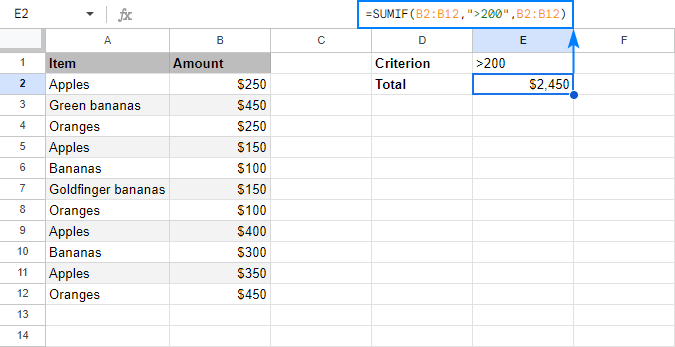Or, you can type the number in some cell, and concatenate the comparison operator with a cell reference:

`=SUMIF(B5:B13, ">"&B1, B5:B13)`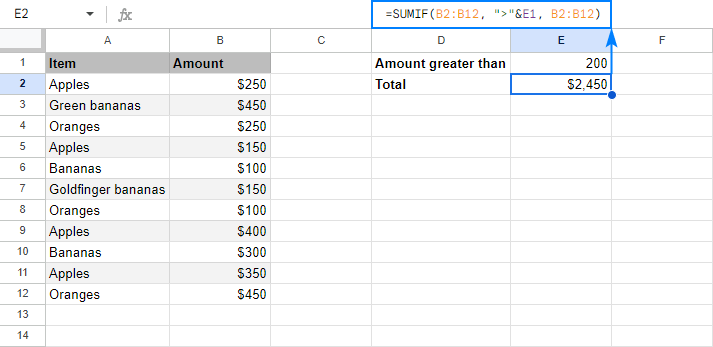You can even input both the comparison operator and number in separate cells, and concatenate those cells: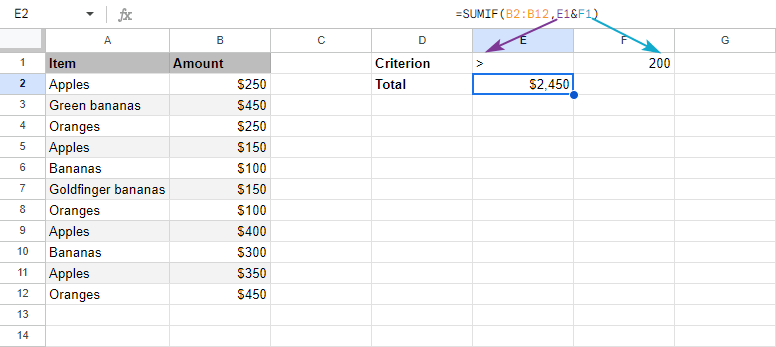In a similar manner, you can use other logical operators such as:

Sum if greater than or equal to 200:

`=SUMIF(B5:B13, ">=200")`

Sum if less than 200:

`=SUMIF(B5:B13, "<200")`

Sum if less than or equal to 200:

`=SUMIF(B5:B13, "<=200")`

#### Sum if equal to

To sum numbers that equal a specific number, you can use the equality sign (=) together with the number or omit the equality sign and include only the number in the criterion argument.

For example, to add up amounts in column B whose quantity in column C is equal to 10, use any of the below formulas:

`=SUMIF(C5:C13, 10, B5:B13)`

or

`=SUMIF(C5:C13, "=10", B5:B13)`

or

`=SUMIF(C5:C13, B1, B5:B13)`

Where B1 is the cell with the required quantity.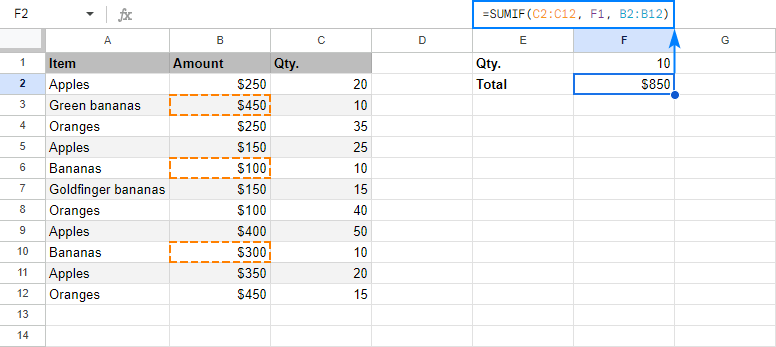#### Sum if not equal to

To sum numbers other than the specified number, use the not equal to operator (<>).

In our example, to add up the amounts in column B that have any quantity except 10 in column C, go with one of these formulas:

`=SUMIF(C5:C13, "<>10", B5:B13)`

`=SUMIF(C5:C13, "<>"&B1, B5:B13)`

The screenshot below shows the result: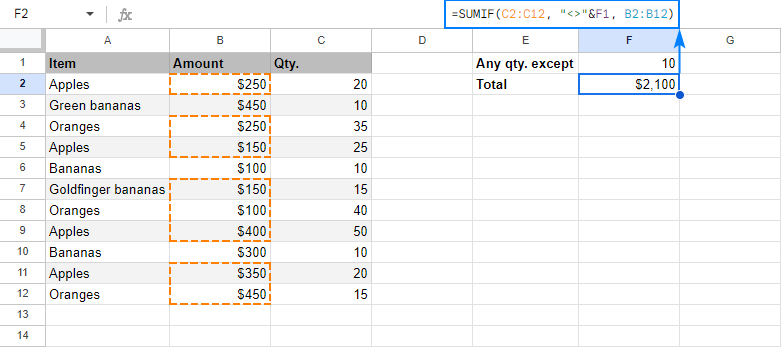### Google Sheets SUMIF formulas for dates

To conditionally sum values based on date criteria, you also use the comparison operators like shown in the above examples. The key point is that a date should be supplied in the format that Google Sheets can understand.

For instance, to sum amounts in B5:B13 for delivery dates prior to 11-Mar-2018, build the criterion in one of these ways:

`=SUMIF(C5:C13, "<3/11/2018", B5:B13)`

`=SUMIF(C5:C13, "<"&DATE(2018,3,11), B5:B13)`

`=SUMIF(C5:C13, "<"&B1, B5:B13)`

Where B1 is the target date: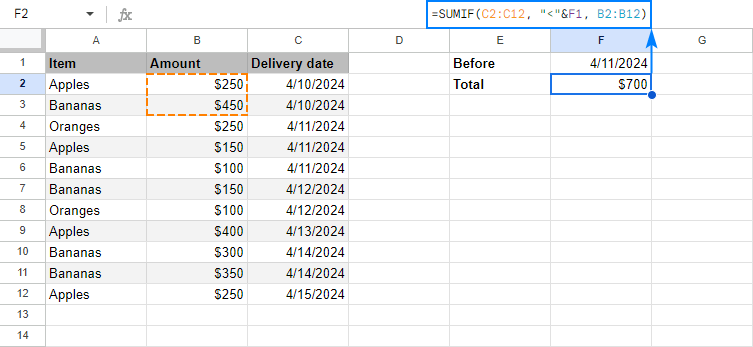In case you want to conditionally sum cells based on today's date, include the TODAY() function in the criterion argument.

As an example, let's make a formula that adds up the amounts for today's deliveries:

`=SUMIF(C5:C13, TODAY(), B5:B13)`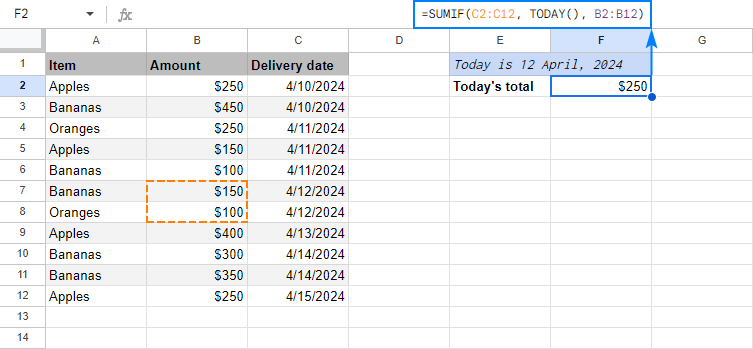Taking the example further, we can find a total of past and future deliveries:

Before today: `=SUMIF(C5:C13, "<"&TODAY(), B5:B13)`

After today: `=SUMIF(C5:C13, ">"&TODAY(), B5:B13)`

### Sum based on blank or non-blank cells

In many situations, you may need to sum values in a certain column if a corresponding cell in another column is or is not empty.

For this, use one of the following criteria in your Google Sheets SUMIF formulas:

#### Sum if blank:

• "=" to sum cells that are completely blank.
• "" to sum blank cells including those that contain zero length strings.

#### Sum if not blank:

• "<>" to add up cells that contain any value, including zero length strings.

For example, to sum the amounts for which the delivery date is set (a cell in column C is not empty), use this formula:

`=SUMIF(C5:C13, "<>", B5:B13)`

To get a total of the amounts with no delivery date (a cell in column C is empty), use this one:

`=SUMIF(C5:C13, "", B5:B13)`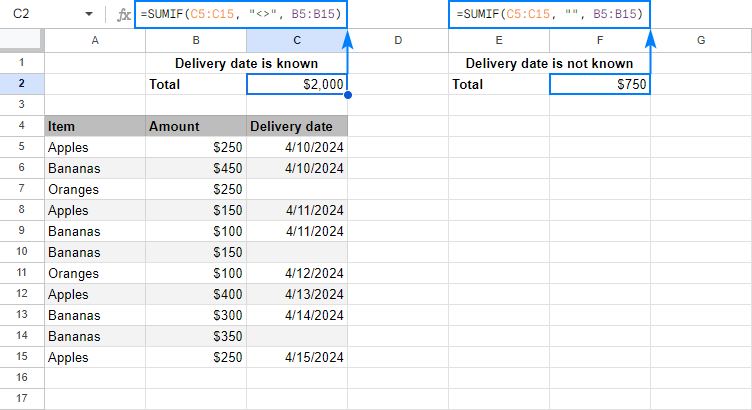### Google Sheets SUMIF with multiple criteria (OR logic)

The SUMIF function in Google Sheets is designed to add up values based on just one criterion. To sum with multiple criteria, you can add two or more SUMIF functions together.

For example, to sum Apples and Oranges amounts, utilize this formula:

`=SUMIF(A6:A14, "apples", B6:B14)+SUMIF(A6:A14, "oranges", B6:B14)`

Or, put the item names in two separate cells, say B1 and B2, and use each of those cells as a criterion:

`=SUMIF(A6:A14, B1, B6:B14)+SUMIF(A6:A14, B2, B6:B14)`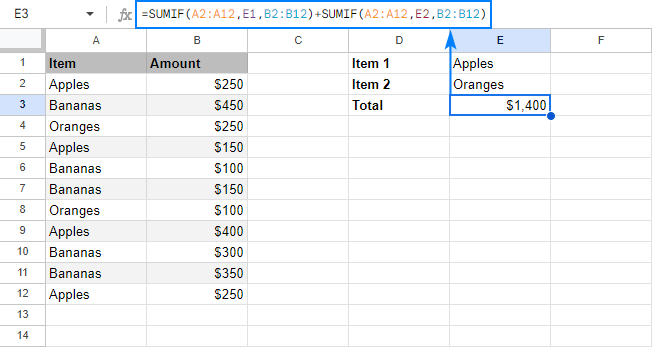Please note that this formula works like SUMIF with OR logical - it sums values if at least one of the specified criteria is met.

In this example, we add values in column B if column A equals "apples" or "oranges". In other words, SUMIF() + SUMIF() works like the following pseudo-formula (not a real one, it only demonstrates the logic!): sumif(A:A, "apples" or "oranges", B:B).

If you are looking to conditionally sum with AND logical, i.e. add up values when all of the specified criteria are met, use the Google Sheets SUMIFS function.

### Google Sheets SUMIF - things to remember

Now that you know the nuts and bolts of the SUMIF function in Google Sheets, it may be a good idea to make a short summary of what you've already learned.

#### 1. SUMIF can evaluate only one condition

The syntax of the SUMIF function allows for only one range, one criterion and one sum_range. To sum with multiple criteria, either add several SUMIF functions together (OR logic) or use SUMIFS formulas (AND logic).

#### 2. The SUMIF function is case-insensitive

If you are looking for a case-sensitive SUMIF formula that can differentiate between uppercase and lowercase characters, use SUMIF in combination with ARRAYFORMULA and FIND as shown in this example.

#### 3. Supply equally sized range and sum_range

In fact, the sum_range argument specifies only the upper leftmost cell of the range to sum, the remaining area is defined by the dimensions of the range argument.

To put it differently, SUMIF(A1:A10, "apples", B1:B10) and SUMIF(A1:A10, "apples", B1:B100) will both sum values in the range B1:B10 because it is the same size as range (A1:A10).

So, even if you mistakenly supply a wrong sum range, Google Sheets will still calculate your formula right, provided the top left cell of sum_range is correct.

That said, it is still recommended to provide equally sized range and sum_range to avoid mistakes and prevent inconsistency issues.

#### 4. Mind the syntax of SUMIF criteria

For your Google Sheets SUMIF formula to work correctly, express the criteria the right way:

• If the criterion includes text, wildcard character or logical operator followed by a number, text or date, enclose the criterion in quotation marks. For example:

`=SUMIF(A2:A10, "apples", B2:B10)`

`=SUMIF(A2:A10, "*", B2:B10)`

`=SUMIF(A2:A10, ">5")`

`=SUMIF(A5:A10, "<>apples", B5:B10)`

• If the criterion includes a logical operator and a cell reference or another function, use the quotation marks to start a text string and ampersand (&) to concatenate and finish the string off. For example:

`=SUMIF(A2:A10, ">"&B2)`

`=SUMIF(A2:A10, ">"&TODAY(), B2:B10)`

#### 5. Lock ranges with absolute cell references if needed

If you plan to copy or move your SUMIF formula at a later point, fix the ranges by using absolute cell references (with the \$ sign) like in SUMIF(\$A\$2:\$A\$10, "apples", \$B\$2:\$B\$10).

This is how you use the SUMIF function in Google Sheets. To have a closer look at the formulas discussed in this tutorial, you are welcome to open our sample SUMIF Google Sheet. I thank you for reading and hope to see you on our blog next week!

## You may also be interested in

1. Ah .. Big thanks to you dear.. Thank you very much//

2. Dang this was intuitive. I was able to use this and got a few things working. I ran into one oddball I think that I can't figure out.

So basically I'm trying to first locate a name in a range. Then I want it to only add up all of the negative numbers and give me that total.

So say that I have this

COL A COL B COL C COL D
3 Steve -5000 Steve